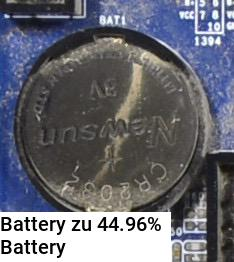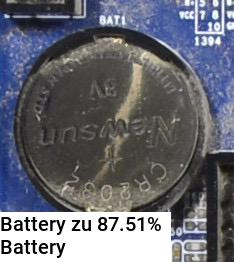# How can my net produce negative outputs when I use ReLU?

Im training an AlexNet and for AlexNets they normally use as activation function the basic ReLU.

Here my AlexNet:

``````class AlexNet(nn.Module):

def __init__(self, num_classes=2):
super(AlexNet, self).__init__()
self.features = nn.Sequential(
nn.ReLU(inplace=True),
nn.MaxPool2d(kernel_size=3, stride=2),
nn.ReLU(inplace=True),
nn.MaxPool2d(kernel_size=3, stride=2),
nn.ReLU(inplace=True),
nn.ReLU(inplace=True),
nn.ReLU(inplace=True),
nn.MaxPool2d(kernel_size=3, stride=2),
)
self.classifier = nn.Sequential(
nn.Dropout(),
nn.Linear(256 * 7 * 7, 4096),
nn.ReLU(inplace=True),
nn.Dropout(),
nn.Linear(4096, 4096),
nn.ReLU(inplace=True),
nn.Linear(4096, num_classes),
)

def forward(self, x):
x = self.features(x)
x = x.view(x.size(0), 256 * 7 * 7)
x = self.classifier(x)
return x
``````

How is it possible that this net structure produces negative outputs ?

As input I got normalize images in 256x256. The images got negative and positive values after normalizing but that should not be the reason why the net outputs negative values. When they are still negative values after the first convolution layer the ReLU should transform all negative values to zero or Im I completely wrong ?

You are right in general, but the last linear layer wight have negative weights resulting in a negative output.

4 Likes

Ah yes of course im stupid. But I have another question. When I want to get the probabilities of my 2 classes I have to apply something like sigmoid on the outputs of my net first and then softmax right?

If you would like to predict only one class, i.e. the probabilities of all classes should sum to one, you should apply `F.softmax(x, dim=1)`.
Otherwise, if multiple classes out your output could be correct, you could apply `F.sigmoid(x)`.

The first approach is more likely. The second approach is used for multi-label classification tasks.

EDIT:
As a side note: I wouldn’t recommend adding `F.softmax` into the model definition, since you would need another `F.log()` outside on the model to apply `nn.NLLLoss`, which could be numerically unstable.
Just return your logits, apply `nn.CrossEntropyLoss` and see your probabilities outside the model with `F.softmax`.

4 Likes

Im not sure if you got me right.

My first task is a binary classification task. And for illustration I want to display the image with the associated probability on it. And therefore I need to apply for example `F.sigmoid()` to the output of the net before I can apply `F.softmax()` because with only softmax I get wired results for the probabilities. (Thats because of the positive and negative outputs of my net that `F.softmax()` can’t handle alone)

And in my next task a have more than 2 classes and want to illustrate the same. I do thats the same way. First sigmoid then softmax.

While training the net for the binary classification I apply sigmoid to the outputs first and then I send them in my loss function which is `F.binary_cross_entropy`. And then I propagate back.

While training the net for the multiple classification. I send the outputs straight into the loss function `nn.CrossEntropyLoss()` because that loss function combines `nn.LogSoftmax()` and `nn.NLLLoss()`. Because of that combination I thought I don’t have to apply another function before I can send the outputs into the loss function.

You see something wrong here ?

Softmax can handle logits, i.e. positive and negative values. In your current code snippet, it seems your model output is of dimension `[batch_size, 2]`, which is a multi-class classification applied on a binary task.
In such a case, you should just apply the softmax to get the probabilities for visualization:

``````logits = torch.randn(4, 2) # your model output
print(logits) # positive and negative values
> tensor([[ 0.0355, -0.6179],
[ 0.3327,  0.0276],
[-1.3194, -0.2547],
[-0.0072,  1.0901]])
prob = F.softmax(logits, dim=1)
print(prob)
> tensor([[ 0.6578,  0.3422],
[ 0.5757,  0.4243],
[ 0.2564,  0.7436],
[ 0.2503,  0.7497]])
``````

As you can see the probabilities sum to one over dim1.
If you would like to use sigmoid and `BCELoss`, your model output should just return `[batch_size, 1]`.

Could you explain your weird results using softmax?

This would most likely work, but is treating your problem as a multi-label classification task, i.e. the BCE formula is applied on each output of your model.

That’s perfectly fine and that’s exactly what I’ve tried to explain before. Sorry, if the explanation was a bit unclear.

2 Likes

Okay I got your point. For the ‘binary’ task when I got you right I should use the `nn.CrossEntropyLoss()` as loss function too because my net outputs is of size `[batch_size, 2]` right ? But strange that I got really good results with`F.sigmoid` + `BCELoss` too.

Thats what I mean with weird. Thats seems not to be what I expect.

``````x = torch.zeros(1,2)
x[0,0] = -3
x[0,1]= 5
out = F.softmax(Variable(x), dim=1)

Variable containing:
0.0003  0.9997
[torch.FloatTensor of size 1x2]
``````

I guess you explained it good but my English is not that good and thats the reason I could not get your point in first place.

Yes, in the general case you would apply `nn.CrossEntropyLoss` on the logits.
Your use case (sigmoid + BCE) might still work, but has additional properties:
your target could also be `[0, 0]`, i.e. no class is found in the sample, or `[1, 1]`, i.e. both classes are valid for this sample.
This would not be possible using `CrossEntropyLoss`.

Your code snippet is expected behavior. Softmax calculates the probabilities based on the “distance” between your logits.
You could write it as:

``````x = torch.tensor([[-3., 5.]])
prob = torch.exp(x) / torch.exp(x).sum()
``````

The logits (input to softmax) aren’t bounded in any way, i.e. they might take really small or large values.
Even negative values would result in a valid probability:

``````x = torch.tensor([[-100., -99.]])
prob = torch.exp(x) / torch.exp(x).sum()
``````
1 Like

Which weighted loss function you would suggest if one of the classes is underrepresented in the training dataset?

I could already reach good results on the multi class task(3 different classes) with `nn.CrossEntropyLoss()` as loss. With good results I mean that the net is in over 90% right with his class prediction. But when I check the probabilities I could see that they are pretty low. With low I mean the average probabilities is around 40-45% for the predicted class. Now I want to try weighted loss here because 1 out of the 3 classes is rly underrepresented and maybe I can fix the issue with the low probabilities like that.

`nn.CrossEntropyLoss` has a `weight` argument, which takes class weights.
If you have an imbalanced dataset, this could help to focus the model on learning the undersampled class.
Note that the per class accuracies of the other classes (majority classes) might suffer from this approach.

Its 25/436/404 without augmentation. But its visually a pretty easy task and thats why I get such a good accuracy on the prediction I think.

You think a weight tensor like [0.8,0.1,0.1] could work?

Or do you have any other suggestion how I can reach higher probabilities for the predictions?

Do you care more about a specific class or is each per class accuracy of equal importance?
Based on the class counts, you could try `weight=torch.tensor([4, 0.23, 0.25])`.
Note that the weights do not have to sum to one. You could also try your weights first.

Did you observe the confusion matrix? Could you post it using the validation dataset?

In the first place I was pretty happy about the fact that my net is pretty good with its predictions but in the second place I checked the probabilities of the predictions. I did not analyzed the probabilities in detail but what I have noticed so far is that the predictions only got around 40-45%. I think I explained that bad therefore I will make I little example.

My test set includes 40 images. When it comes to class prediction my net got nearly all of the 40 images right. But when I check the probabilities the decisions are based on its often something like `[0.4,0.36,0.34]`. I mean the net is nearly every time right with its predictions but the decisions are pretty close. You know what I mean? Or do I maybe just need to train more epochs that the net can consolidate his predictions? So far I train the net only 15 epochs.

No I did not observe the confusion matrix. I just checked what a confusion matrix is and I try to make a confusion matrix by hand tomorrow.

You could observe the loss of your validation data and stop the training at the lowest point, i.e. when the model starts to overfit to your dataset. This could stabilize your probabilities a bit.

OK, let me know, if you get stuck computing the confusion matrix.

I coded my own little confusion matrix function now.

``````def confusion_matrix_maker(file_name):
pos1 = 0
pos2 = 0

img = Image.open(img_working + file_name)
img_eval_tensor = transform(img)
img_eval_tensor.unsqueeze_(0)
data = Variable(img_eval_tensor)

if 'C1' in file_name:
pos1 = 0
elif 'C2' in file_name:
pos1 = 1
elif 'C3' in file_name:
pos1 = 2

net2.eval()
output = net2(data)

if output.data.max(1, keepdim=True).numpy() ==0:
pos2 = 0
elif output.data.max(1, keepdim=True).numpy() ==1:
pos2 = 1
elif output.data.max(1, keepdim=True).numpy() ==2:
pos2 = 2

confusion_matrix[pos1,pos2]+=1
``````

Thats the output:

`````` 84   3   0
2  78   0
0   0   6
[torch.FloatTensor of size 3x3]
``````

But I think you got not my problem. Below is one output of my result visualization function. He predicted the class battery correct but the probability is very low. First I applied `F.sigmoid()` and then `F.softmax()` to get these probabilities. The confusion matrix above is by the way from the same test dataset with the same net where this pic came from.The next picture is out of the same result visualization function and with the same net but here I applied only `F.softmax()` to the outputs of the net.The second method with only `F.softmax()` feels not right for me because he totally underrates the big negative value and that seems not right for me or im I wrong here ? Below is the net output and the second tensor is `F.softmax()` applied on the output for the picture above.

``````Variable containing:
-4.6320  1.3062  3.2554
[torch.FloatTensor of size 1x3]

Variable containing:
0.0003  0.1246  0.8751
[torch.FloatTensor of size 1x3]
``````

It’s rather on the contrary!
You should apply softmax on the raw logits without squashing them using a sigmoid. If you apply a sigmoid before, your probabilities will end up closer together.
Have a look at the probability interpretation of softmax from Karpathy’s blog post.

In the first post in this topic I mentioned that my input got a lot of negative values after normalization.

As input I got normalize images in 256x256. The images got negative and positive values after normalizing but that should not be the reason why the net outputs negative values. When they are still negative values after the first convolution layer the ReLU should transform all negative values to zero or Im I completely wrong ?

Do the negative values hinder the training process? Should I try to rescale it into something between`[0,1]` ?

It shouldn’t. I assume you are normalizing you data, i.e. you are whitening the signal.
This is a standard procedure and your training should benefit from a white signal.

Yes, I normalize the data with the mean and standard deviation of the training set.

Should be alright. Your confusion matrix looks good to me.
You could speed up the code a bit:

``````model.eval()
conf_mat = torch.zeros(3, 3)